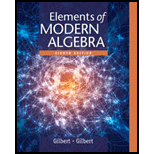# Write out the matrix that matches the given description. A = [ a i j ] 3 × 2 w i t h a i j = 2 i − j . A = [ a i j ] 4 × 2 w i t h a i j = ( − 1 ) i j . B = [ b i j ] 2 × 4 w i t h b i j = ( − 1 ) i + j . B = [ b i j ] 3 × 4 w i t h b i j = 1 i f i &lt; j a n d b i j = 0 i f i ≥ j . C = [ c i j ] 4 × 3 w i t h c i j = i + j i f i ≥ j a n d c i j = 0 i f i &lt; j . C = [ c i j ] 4 × 3 w i t h c i j = 0 i f i ≠ j a n d c i j = 1 i f i = j### Elements Of Modern Algebra

8th Edition
Gilbert + 2 others
Publisher: Cengage Learning,
ISBN: 9781285463230### Elements Of Modern Algebra

8th Edition
Gilbert + 2 others
Publisher: Cengage Learning,
ISBN: 9781285463230

#### Solutions

Chapter
Section
Chapter 1.6, Problem 1E
Textbook Problem

## Expert Solution

### Want to see the full answer?

Check out a sample textbook solution.See solution

### Want to see this answer and more?

Experts are waiting 24/7 to provide step-by-step solutions in as fast as 30 minutes!*

See Solution

*Response times vary by subject and question complexity. Median response time is 34 minutes and may be longer for new subjects.